Design tidal turbine or wind turbine software heliciel
Tutorial tidal turbine design
We will discuss here the design of a tidal turbine in open water with héliciel . The tidal turbine differs from the hydraulic turbine Kaplan, because it is not in a penstock. The study of the hydraulic turbine Kaplan in penstock, is detailed in the pages of hydropower.
• See also, for general information on the turbines, the general section of turbines.
• The tidal turbines are of the same nature as the wind turbines, Tutorial on wind turbines should be consulted to master the concept prerequisite, about speed, and optimum operating point, of the rotor energy capture.
The specificity of the tidal turbines is mainly in mastering the problems of cavitation ... We will see how to manage and visualize the cavitation our tidal turbine. Pour commencer , To begin, open a tidal turbine model using the File menu: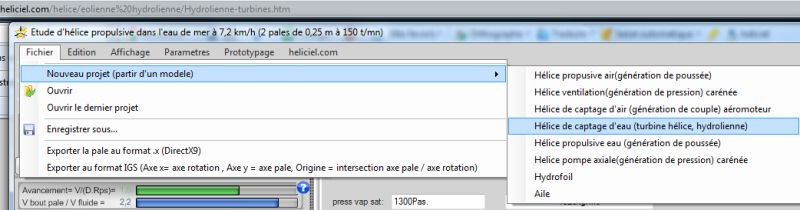• The tidal turbine project by default, is a study of tidal turbine Sea Gen 16 meters in diameter in a fluid stream of 2.4 m / sec. We will use this tidal turbine Sea gen for our case study:• Rotor diameter = 16 meters
• Fluid velocity at design point = 2.4 m/sec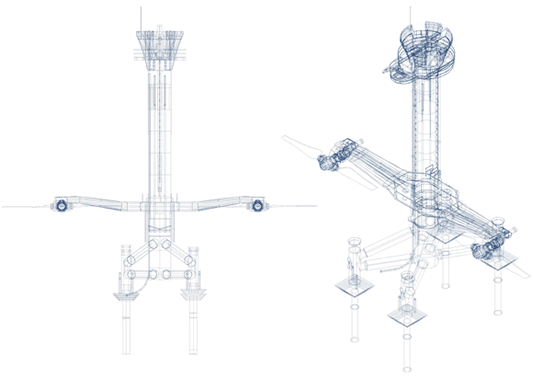• The Operating point for which the geometry of the model has been optimized ("design Point") is visible in the tab 1.3 Operating point:
• fluid velocity 2.4 m/sec,
• rotation speed 18.2 rpm
• The dimensional parameters of the blade are shown in the tab: 2.1 "Blade Dimensions":
• The rotor is 16 meters in diameter, so the blade tip radius is 8000 mm..
• The radius at the blade root is estimated at 17% (1360mm)
• The chord at the blade root is estimated at 2000 mm
• The chord at the blade tip is estimated at 1000 mm
• distribution of intermediate chords has an equation of Chord=(2,510035E-05) x r²+(-0,3855419)xr+2477,912
• In the tab 2.2 the constant profil low is naca643418

see the 3D model of the tidal turbine
• The shaft power is about 0.78 MW , This tidal turbine, is scheduled for a  1.2MW power with2 rotors , if we take our model: 0.78x2=1.56 MW at the shaft. By applying a coefficient of performance 0.77 for the transmission ratio, and the generator , we find the manufacturer data..
The term "operating point" and "point design" should be differentiated::
• The operating point is for a given fluid velocity, the real speed of rotation of the tidal turbine. The real speed of rotation depends on the balance between the torque produced by the tidal rotor and the resistant torque of the generator and various organs. If the load torque is smaller than the torque of the rotor,The propeller rotates faster, until the torque is equal to the load torque. If the load torque is zero (gearbox broken generatrice without load ...) the speed of the tidal turbine rise up to the runaway speed, Which corresponds to the velocity where the rotor produces a zero or negative torque. To know this runaway speed with Heliciel, multiple analysis is used (Optimize tab) and multipoint analysis "off design" is launched ,Parameter varying is the "rotation speed". This produced the torque curve as a function of the speed variation. Here we see that our tidal turbine possesses a 37 rpm runaway speed , And that, at this speed, the thrust will be 750 000 Newtons , Hopefully the engineers calculated the resistance of the support for this scenario ......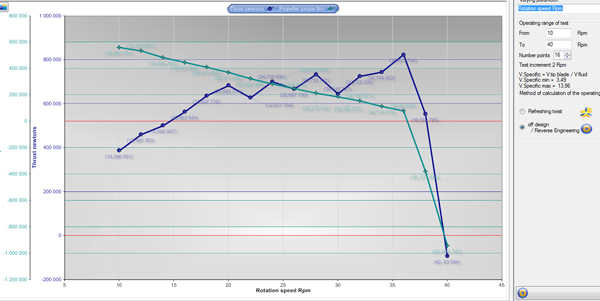• The design point is the combination velocity rotation, fluid velocity at which the twisting of the blades has been optimized. All other parameters of rotation speed or velocity of fluid, will produce lower efficiency.

• Cavitation Risk analysis of the tidal turbine. Pressures displayed in the result area are given for each area of the blades and are calculated from 3 different way:
• An average pressure, corresponding to the lift spread over the entire upper surface,
• a concentrated pressure is calculated on the region between the lift point and the leading edge
• And "pressure drag", calculated according to the projected drag on the thickness of the element.
This allows us to distinguish three types of cavitation (see cavitation). The cavitation threshold is marked by a red line :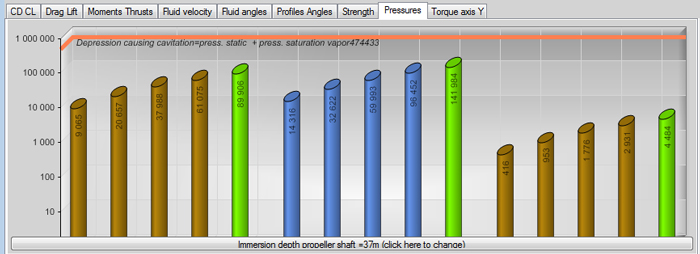• Another way to visualize the pressure is to color the blade according a color code by using the function "show pressure" ..: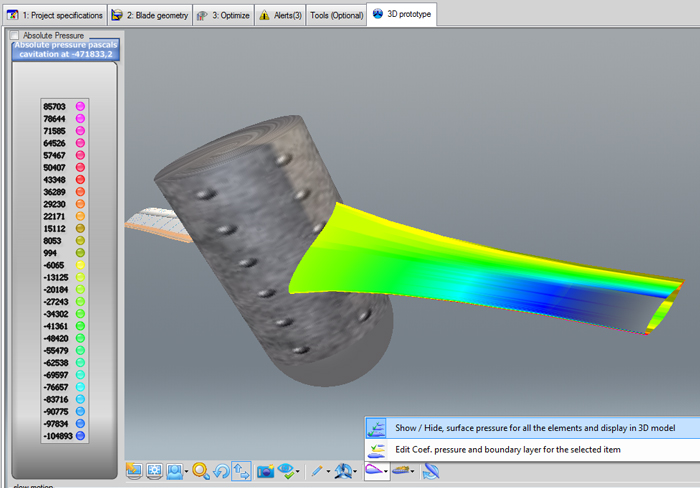This is the end of this little tutorial software modeling and calculation heliciel on the rotor design of the tidal turbines, which should put you on the right track to create tidal turbines or recovery of energy on board ships, for example. .......